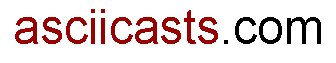#ASCIIcasts

## 2: Dynamic find_by Methods

(view original Railscast)

This is a really useful way to do a `Find` in Rails. Below is a `Task` model that searches for tasks that haven’t been completed (i.e. the `complete` column is `false`).

``` class TaskController < ApplicationController def incomplete @tasks = Task.find(:all, :conditions => ['complete = ?', false]) end def last_incomplete @task = Task.find(:first, :conditions => ['complete =?', false], :order => 'created_at DESC') end end ```

There is a better way to achieve this with `find_by_all`. Just replace

`@tasks = Task.find(:all, :conditions => ['complete = ?', false])`

with

`@tasks = Task.find_all_by_complete(false)`

If you just want to find one `Task` then use `find_by`, so to find the latest incomplete task the line

`@task = Task.find(:first, :conditions => ['complete =?', false], :order => 'created_at DESC')`

becomes

`@task = Task.find_by_complete(false, :order => 'created_at DESC')`

The `find_by` method takes the `order` parameter just like the `find` method does.